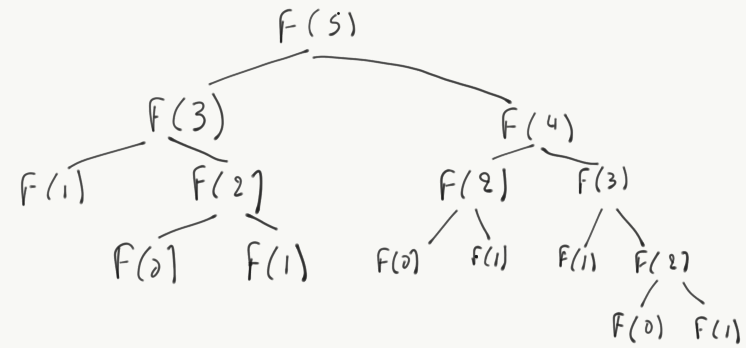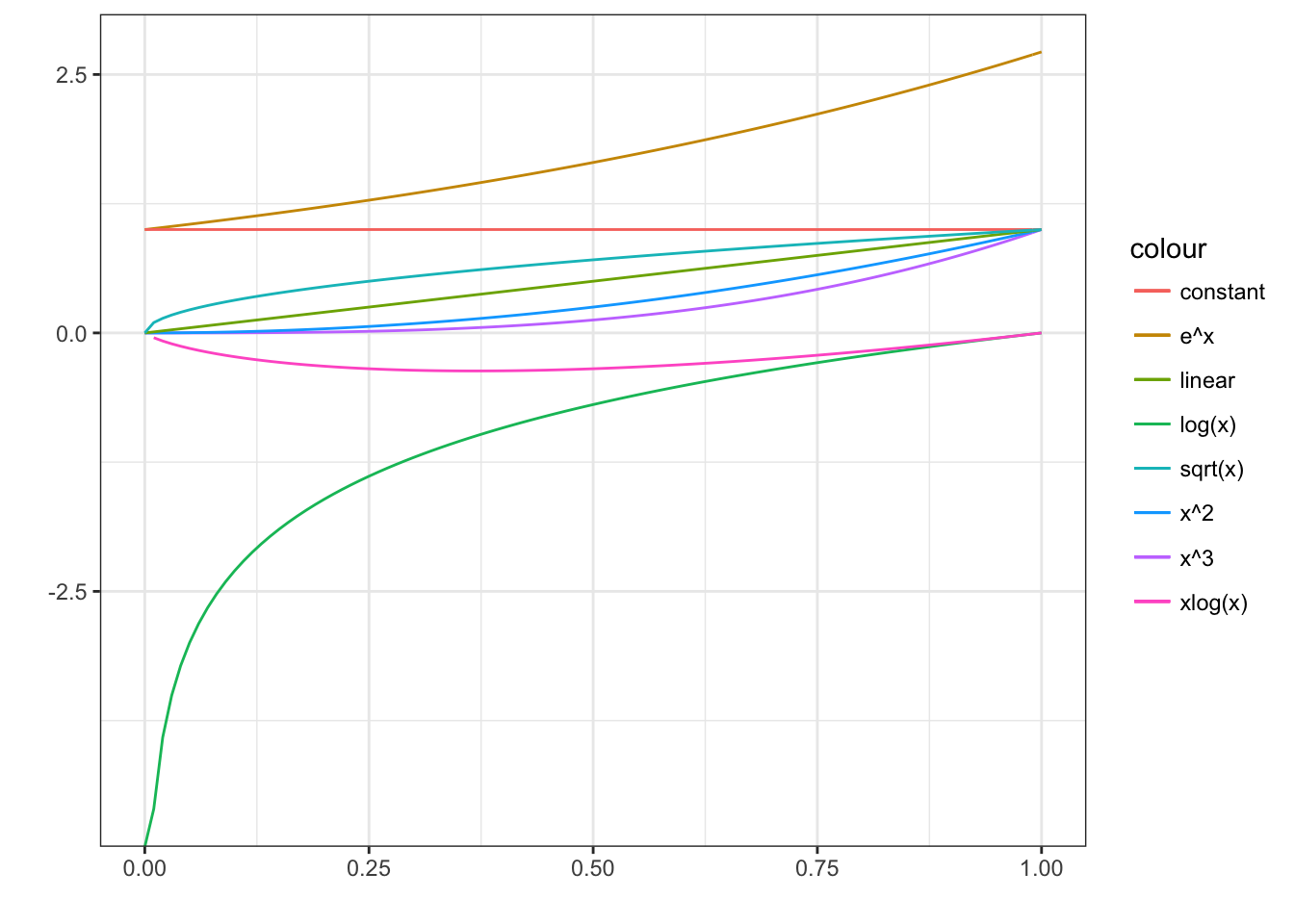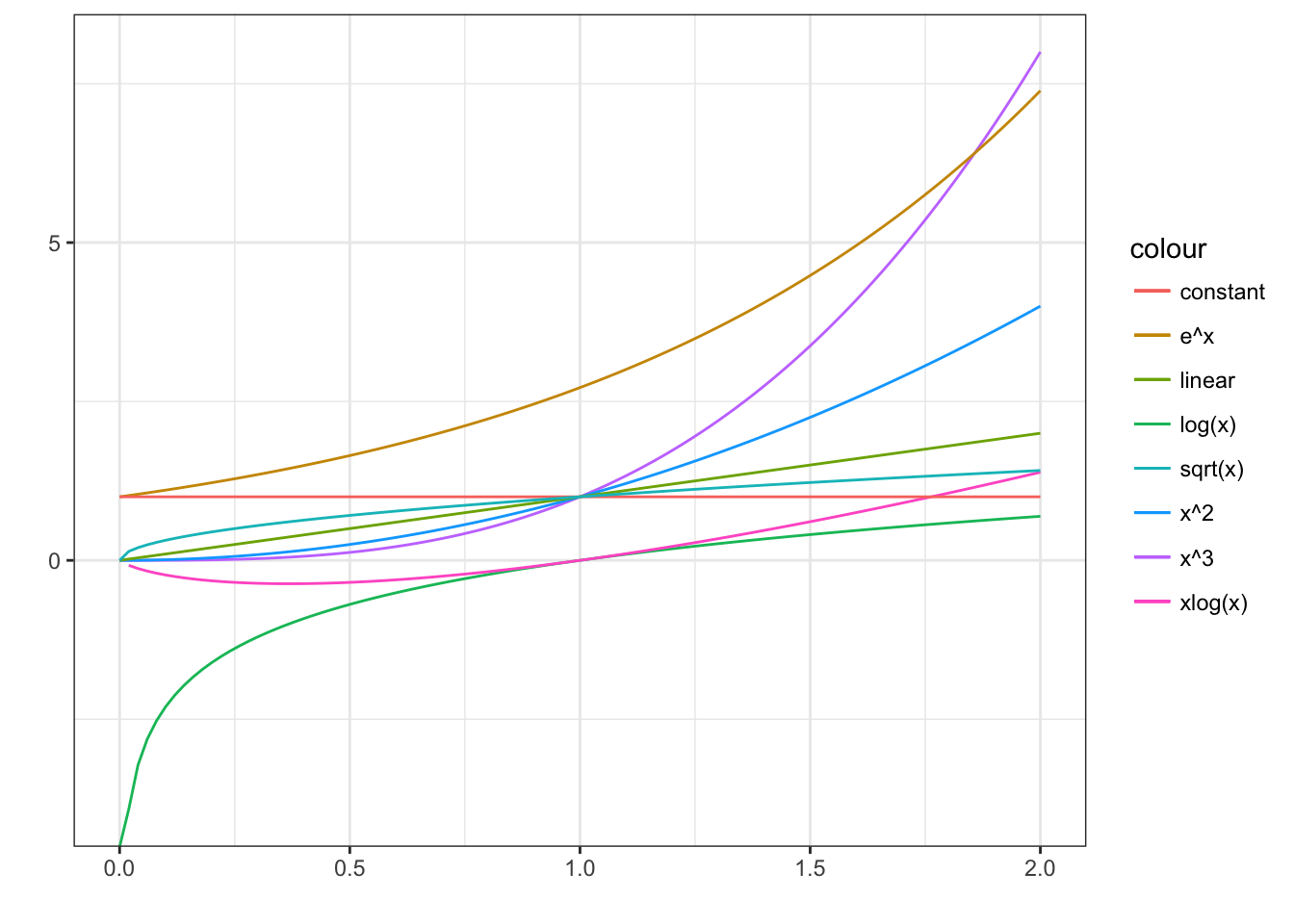# Algorithm Analysis

## Algorithm analysis

Algorithm analysis is the theoretical study of program performance and resource usage.

D: What resources do programs consume?

• CPU time
• Memory and disk
• Network I/O

Q: Why is algorithm analysis important?

Because it allows us to determine what is possible to do given a problem.

## A simple (?) algorithm

def fib(x):
if x == 0: return 0
elif x == 1: return 1
else: return fib(x - 2) + fib(x - 1)

D: How many steps will this algorithm need to make to calculate fib(5)?Fib(5) call tree

## Analysing fib

What we observe is that fib takes a number of steps that is proportional to its argument.

• fib(2) requires 2 steps
• fib(3) requires steps(fib(2)) + 1 = 3 steps
• fib(4) requires steps(fib(3)) + steps(fib(2)) + 1 = 6 steps

The question that algorithm analysis will enable us answer is:

How many steps does fib(n) require?

## Kinds of algorithm analyses

• Worst-case: $$T(n)$$ The maximum steps/time/memory taken by an algorithm to complete the solution for inputs of size $$n$$

• Average-case: The expected time over all inputs with the same characteristics (e.g. size).

• Best-case: The performance in ideal situations. Rarely used.

When analysing algorithmic performance, we ignore computational resources ( e.g. CPU speed); we only care about the growth of $$T(n)$$ as $$n \rightarrow \infty$$

This is called the asymptotic behaviour

## $$\Theta$$ notation

The $$\Theta$$ notation defines its exact asymptotic beviour of a function $$f(n)$$ by restricting its upper and lower bounds in terms of another function:

$$f(n) = \Theta(g(n))$$ means that: $$\forall n > n_0: \exists c_1 > 0, c_2 > 0, n_0:$$ $$0 \le c_1 g(n) \le f(n) \le c_2 g(n)$$

This means that there exist positive constants $$c_1$$, $$c_2$$, and $$n_0$$ such that if $$f(n)$$ is $$\Theta(g(n))$$, then $$f(n)$$ execution steps/time are bound by the equation above.

In practice, this means there exists a value $$n_0$$ such as a $$\Theta(n^2)$$ algorithm will behave worse that $$\Theta(n)$$ algorithm

## $$O$$ notation

Most times we only care about the worse case behaviour. This is what $$O$$ defines:

$$f(n) = O(g(n))$$ means that:

$$\forall n > n_0, \exists c > 0, n_0 > 0: 0 \le f(n) \le cg(n)$$

Q: Is $$2n^2 = O(n^3)$$?

We take $$c = 1$$ and $$n_0 = 2$$ and replace in the “constructor:”

$$0 \le 2n^2 \le n^3$$. The equation holds $$\forall n$$

This also means that we can easily overestimate our compexity scale; we need to be precise.

## Asymptotic growth

Asymptotic growth analysis allows to estimate the (usually, upper) bounds of functions as a function of problem size.

It also enables us to compare algorithm implementations: an $$O(n^2)$$ algorithm is always worse than an $$O(log(n))$$ one.

## Math growth functions

We usually determine the asymptotic growth in terms of the following functions. Each one is asymptotically better than the following:

1. $$f(x) = n$$ (constant function)
2. $$f(x) = log(x)$$ (logarithmic function)
3. $$f(x) = sqrt(x)$$ (square root function)
4. $$f(x) = x$$ (linear function)
5. $$f(x) = nlog(n)$$ (log-linear function)
6. $$f(x) = x^2$$ (quadratic function)
7. $$f(x) = x^3$$ (cubic function)
8. $$f(x) = a^x, \forall a > 1$$ (exponential function)

## Function growth examples

Growth of important functions for $$x \in (0, 1)$$Growth of the same functions for $$x \in (0, 2)$$Growth of the same functions for $$x \in (0, 5)$$